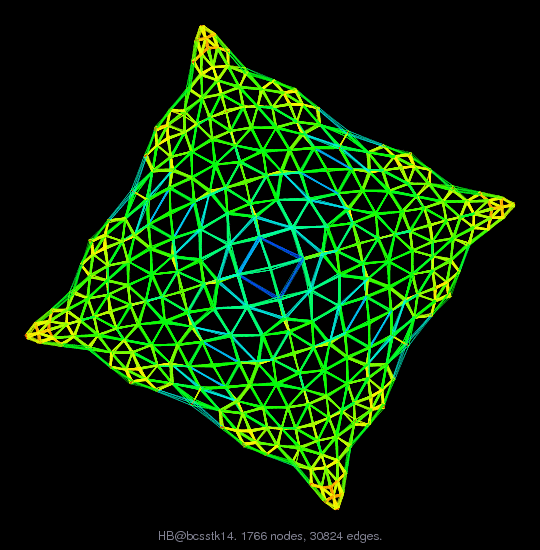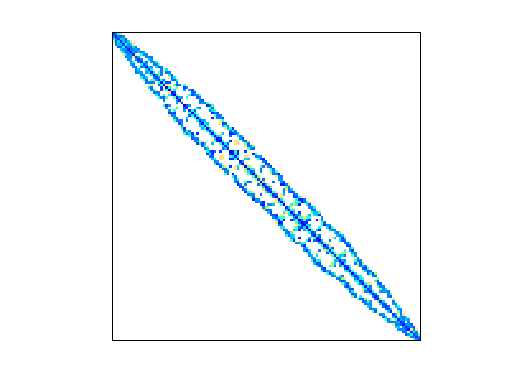Matrix: HB/bcsstk14

Description: S STIFFNESS MATRIX - ROOF OF OMNI COLISEUM, ATLANTA(undirected graph drawing)• Matrix group: HB
• download as a MATLAB mat-file, file size: 359 KB. Use UFget(36) or UFget('HB/bcsstk14') in MATLAB.

 Matrix properties number of rows 1,806 number of columns 1,806 nonzeros 63,454 structural full rank? yes structural rank 1,806 # of blocks from dmperm 41 # strongly connected comp. 41 explicit zero entries 0 nonzero pattern symmetry symmetric numeric value symmetry symmetric type real structure symmetric Cholesky candidate? yes positive definite? yes

 author M. Will editor I. Duff, R. Grimes, J. Lewis date 1985 kind structural problem 2D/3D problem? yes

 Ordering statistics: result nnz(chol(P*(A+A'+s*I)*P')) with AMD 109,078 Cholesky flop count 9.1e+06 nnz(L+U), no partial pivoting, with AMD 216,350 nnz(V) for QR, upper bound nnz(L) for LU, with COLAMD 184,239 nnz(R) for QR, upper bound nnz(U) for LU, with COLAMD 386,801

 SVD-based statistics: norm(A) 1.19232e+10 min(svd(A)) 0.999998 cond(A) 1.19232e+10 rank(A) 1,806 sprank(A)-rank(A) 0 null space dimension 0 full numerical rank? yes

 singular values (MAT file): click here SVD method used: s = svd (full (A)) ; status: ok